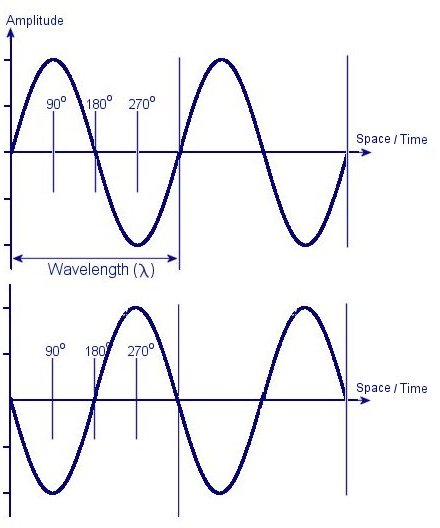WOOCS 1.2.7.1
01270 747 008 (UK)

Properties of Waves

Physical quantities can be placed in one of two categories: Scalar quantities and Vector quantities. Scalars are quantities, which have magnitude only, such as mass, temperature, or electric charge. They can be fully expressed in one value, i.e. the temperature of a room is 18ºC. Vectors, such as velocity and force have two components (magnitude & direction) associated with them. For example the magnitude of a force can be expressed as 10 Newton’s, but to fully express this, its vector component must be expressed too. This could be a force of 10 Newtons, acting vertically at 90º to the ground. The table below should help you see the difference.

 Scalar Quantities Vector Quantities mass force charge velocity temperature momentum speed acceleration distance displacementAn electromagnetic (EM) wave can be described using vectors, as it has both magnitude and directional components. It is a transverse wave, which means it vibrates at right angles to the direction in which it travels. When two or more such waves meet they can interact in a variety of ways. If two waves meet, and are of the same frequency, amplitude and phase, then they will constructively interfere with each other to produce a wave with twice the amplitude. If the two waves met and were out of phase by 180º then they would destructively interfere and therefore cancel each other out. The diagram below is a graphical representation of two EM waves that are 180 degrees out of phase from each other.

The canceling effect witnessed when two waves destructively interfere with each other is a local phenomenon only. This means that the interference only occurs in a small area where the waves meet. The waves will not be stopped, and they will continue on their original paths. In the case of a continuous beam of EM waves or photons an interference pattern will be produced

The laws of thermodynamics tell us that energy can not be destroyed, but these two waves, both carrying energy, seem to to briefly disappear when they interfere. To understand what is actually happening we must look at the energy in a wave as it vibrates. This is covered in the next section.

Next Page: Standing Waves
Previous Page: Electromagnetic Waves

1.2.